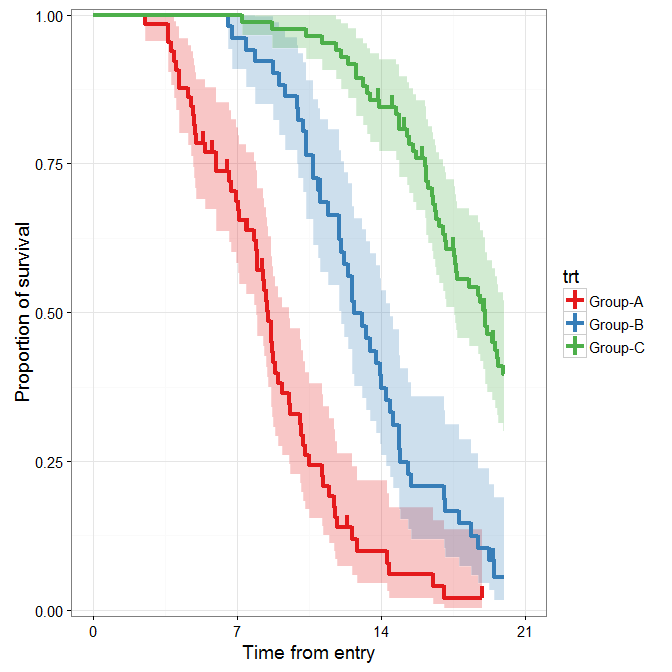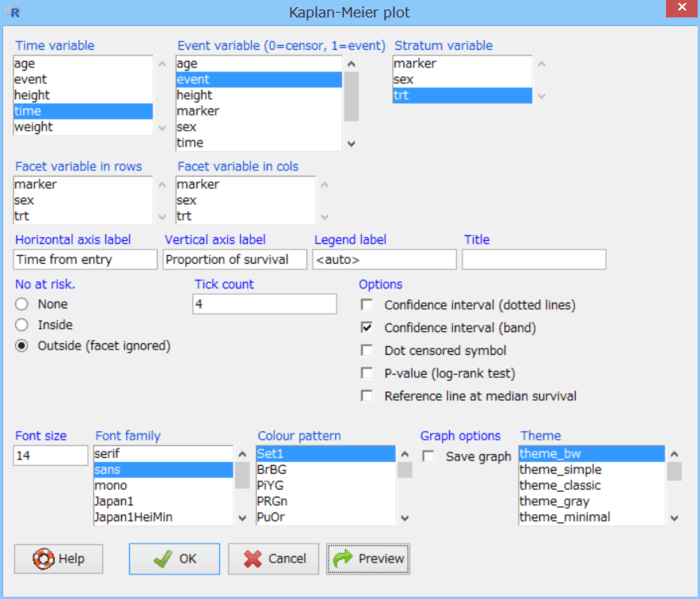Want to share your content on R-bloggers? click here if you have a blog, or here if you don't.

New version (v0.2-3) of ‘RcmdrPlugin.KMggplot2’ (an Rcmdr plug-in; a GUI for ‘ggplot2’) released.

#### NEWS

##### Changes in version 0.2-3 (2015-12-30)
• New geom_stepribbon().
• Pointwise confidence intervals of Kaplan-Meier plots with band (Thanks to Dr. M. Felix Freshwater).
• Notched box plots (Thanks to Dr. M. Felix Freshwater).
• Stratified (colored) box plots (Thanks to Dr. M. Felix Freshwater).
##### Changes in version 0.2-2 (2015-12-22)
• New ggplot2’s theme (theme_linedraw, theme_light).
• New ggthemes’s themes (theme_base, theme_par).
• Fixed a bug was caused by new ggplot2’s theme.
• Fixed a bug related to ggthemes 3.0.0.
• Fixed a bug related to windows fonts.
• Fixed a bug related to file saving.
• Added a vignette for dataset requirements to make Kaplan-Meier plot.
• Added a vignette for extrafont.

#### `geom_stepribbon()`

The `geom_stepribbon` is an extension of the `geom_ribbon`, and is optimized for Kaplan-Meier plots with pointwise confidence intervals or a confidence band.

##### Usage
```geom_stepribbon(mapping = NULL, data = NULL, stat = "identity",
position = "identity", na.rm = FALSE, show.legend = NA,
inherit.aes = TRUE, kmplot = FALSE, ...)
```

• `kmplot`
• If `TRUE`, missing values are replaced by the previous values. This option is needed to make Kaplan-Meier plots if the last observation has an event, in which case the upper and lower values of the last observation are missing. This processing is optimized for results from the `survfit` function.

Other arguments are the same as the `geom_ribbon`.

##### Example
```require("ggplot2")
data(dataKm, package = "RcmdrPlugin.KMggplot2")

.df <- na.omit(data.frame(x = dataKm\$time, y = dataKm\$event, z = dataKm\$trt))
.df <- .df[do.call(order, .df[, c("z", "x"), drop = FALSE]), , drop = FALSE]
.fit <- survival::survfit(
survival::Surv(time = x, event = y, type = "right") ~ z, .df)
.fit <- data.frame(x = .fit\$time, y = .fit\$surv, nrisk = .fit\$n.risk,
nevent = .fit\$n.event, ncensor= .fit\$n.censor, upper = .fit\$upper,
lower = .fit\$lower)
.df <- .df[!duplicated(.df[,c("x", "z")]), ]
.df <- .fit <- data.frame(.fit, .df[, c("z"), drop = FALSE])
.df <- .fit <- rbind(unique(data.frame(x = 0, y = 1, nrisk = NA, nevent = NA,
ncensor = NA, upper = 1, lower = 1, .df[, c("z"), drop = FALSE])), .fit)
.cens <- subset(.fit, ncensor == 1)

ggplot(data = .fit, aes(x = x, y = y, colour = z)) +
RcmdrPlugin.KMggplot2::geom_stepribbon(data = .fit,
aes(x = x, ymin = lower, ymax = upper, fill = z), alpha = 0.25,
colour = "transparent", show.legend = FALSE, kmplot = TRUE) +
geom_step(size = 1.5) +
geom_linerange(data = .cens, aes(x = x, ymin = y, ymax = y + 0.02),
size = 1.5) +
scale_x_continuous(breaks = seq(0, 21, by = 7), limits = c(0, 21)) +
scale_y_continuous(limits = c(0, 1), expand = c(0.01, 0)) +
scale_colour_brewer(palette = "Set1") +
scale_fill_brewer(palette = "Set1") +
xlab("Time from entry") +
ylab("Proportion of survival") +
labs(colour = "trt") +
theme_bw(base_size = 14, base_family = "sans") +
theme(legend.position = "right")
```#### Pointwise confidence intervals of Kaplan-Meier plots with band#### Notched box plots and stratified (colored) box plots